## 一、案例分析

• 1.挂载SD卡
• 2.获取温湿度数据
• 3.在创建SD卡中创建文件，保存数据
• 4.将异常温湿度数据保存到SD文件中

## 二、系统优化

### 1.SD热插处理

``````void FlieSystem_entry(void *parameter)
{
static rt_err_t result;

rt_device_t dev;

while(1)
{
dev = rt_device_find("sd0");

if (dev != RT_NULL)
{
if (dfs_mount("sd0", "/", "elm", 0, 0) == RT_EOK)
{
rt_kprintf("SD mount to / success\n");
break;
}
else
{
rt_kprintf("SD mount to / failed\n");
}
}
}
}

static int FileSystemInit(void)
{
//创建sd线程

{
}
}

INIT_ENV_EXPORT(FileSystemInit);``````

### 2.温湿度数据发送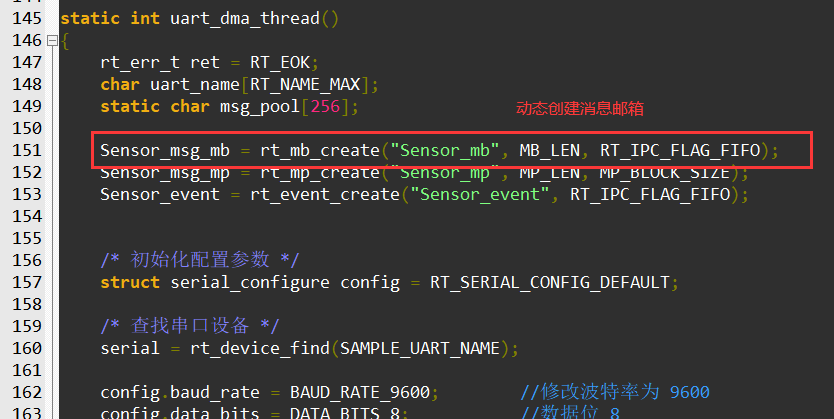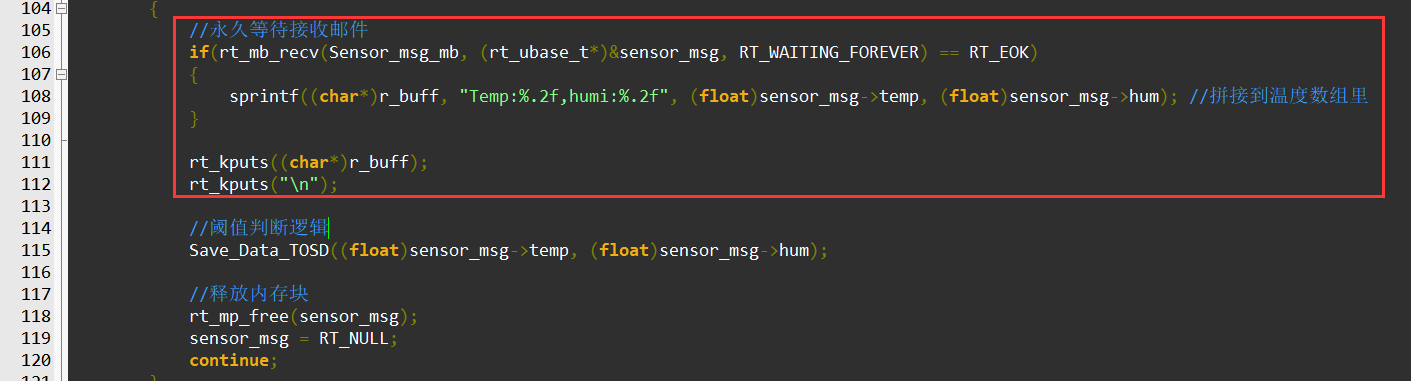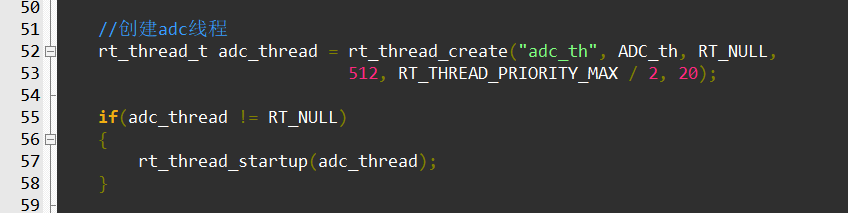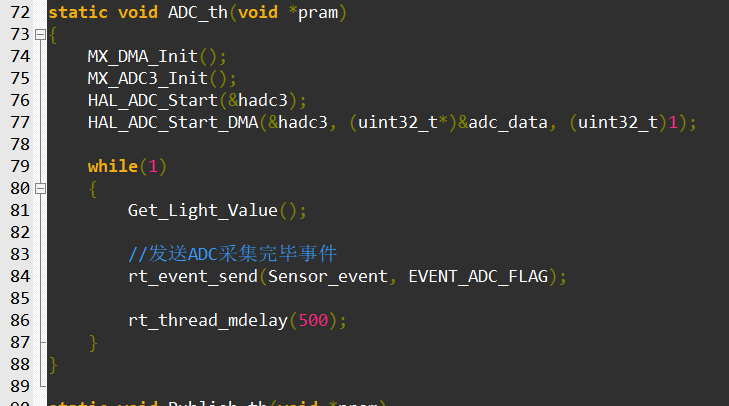## 三、案例实战

### 1.文件的读写操作

``````//保存数据到SD
void Sensor_DataTo_SD(char* buff)
{
/* 以创建和读写模式打开 /text.txt 文件，如果该文件不存在则创建该文件 */
FILE *recvdata_p0;
recvdata_p0 = fopen("/Sensor_Data.csv", "a+");
if (recvdata_p0 != RT_NULL)
{
fputs(buff, recvdata_p0);
fputs("\n", recvdata_p0);
fclose(recvdata_p0);
}
}

//从SD读取信息
{
FILE *fp;
char buffer;
fp = fopen("/Sensor_Data.csv", "r");
if (fp != RT_NULL)
{
rt_kprintf("%s", buffer);
fclose(fp);
}
}``````

### 2.温度阈值判断逻辑

``````void Save_Data_TOSD(float data1, float data2)
{
if(data1 >= HIGHT_TEMPVALUE)
{
detect_logic.T_Count_Alarm++;
}
else
{
detect_logic.T_Count_Alarm--;
if(detect_logic.T_Count_Alarm <= 0)
detect_logic.T_Count_Alarm = 0;
}
if(detect_logic.T_Count_Alarm >= MAX_COUNTER)
{
detect_logic.T_Count_Alarm = MAX_COUNTER;
rt_kprintf("温度超过标准！%d\n",detect_logic.T_Count_Alarm);
sprintf((char*)detect_logic.Alarm_buff, "Temp:%.2f,humi:%.2f", data1, data2); //拼接到温度数组里
//保存异常数据到SD卡
Sensor_DataTo_SD((char*)detect_logic.Alarm_buff);
}
//
if(data2 >= HIGHT_HUMIVALUE)
{
detect_logic.H_Count_Alarm++;
}
else
{
detect_logic.H_Count_Alarm--;
if(detect_logic.H_Count_Alarm <= 0)
detect_logic.H_Count_Alarm = 0;
}

if(detect_logic.H_Count_Alarm >= MAX_COUNTER)
{
detect_logic.H_Count_Alarm = MAX_COUNTER;
rt_kprintf("湿度超过标准！%d\n",detect_logic.H_Count_Alarm);
sprintf((char*)detect_logic.Alarm_buff, "Temp:%.2f,humi:%.2f", data1, data2); //拼接到温度数组里
//保存异常数据到SD卡
Sensor_DataTo_SD((char*)detect_logic.Alarm_buff);
}
}``````

### 3.利用事件进行线程同步

#### 3.1、事件的介绍

• 事件只与线程相关，事件间相互独立：每个线程可拥有 32 个事件标志，采用一个 32 bit 无符号整型数进行记录，每一个 bit 代表一个事件；

• 事件仅用于同步，不提供数据传输功能；

• 事件无排队性，即多次向线程发送同一事件 (如果线程还未来得及读走)，其效果等同于只发送一次。

#### 3.2、事件在系统中的使用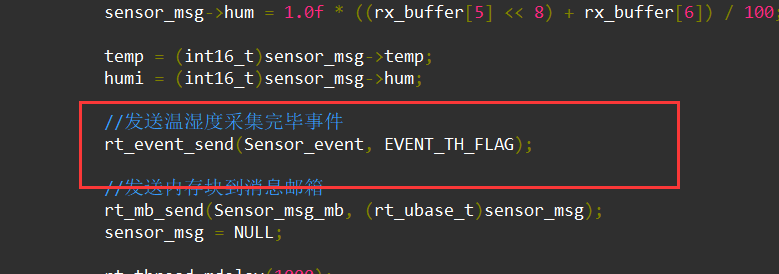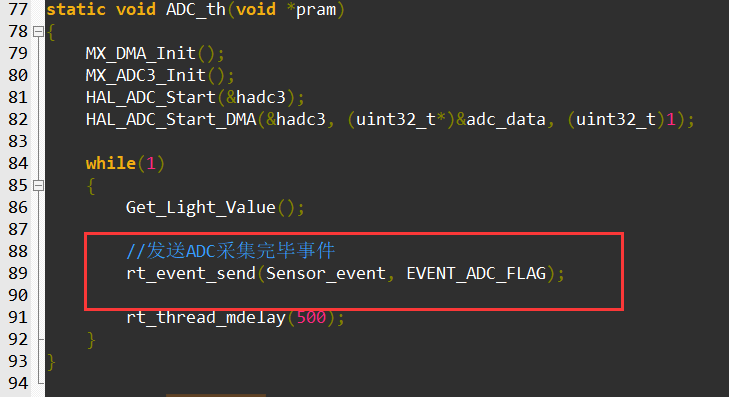### 4.接收线程处理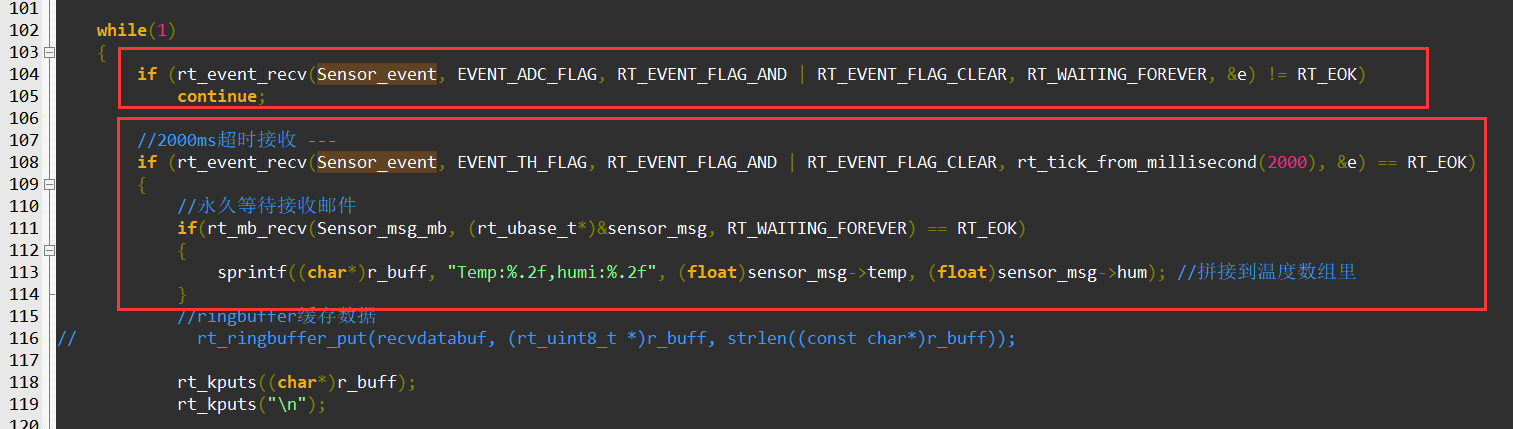``````static void Publish_th(void *pram)
{
//传感器数据结构体
Sensor_msg sensor_msg;
static rt_uint32_t e;
static char r_buff;

while(1)
{
if (rt_event_recv(Sensor_event, EVENT_ADC_FLAG, RT_EVENT_FLAG_AND | RT_EVENT_FLAG_CLEAR, RT_WAITING_FOREVER, &e) != RT_EOK)
continue;

//5s等待接收邮件
if(rt_mb_recv(Sensor_msg_mb, (rt_ubase_t*)&sensor_msg, rt_tick_from_millisecond(5000)) == RT_EOK)
{
sprintf((char*)r_buff, "Temp:%.2f,humi:%.2f", (float)sensor_msg->temp, (float)sensor_msg->hum); //拼接到温度数组里
rt_kputs((char*)r_buff);
rt_kputs("\n");

//阈值判断逻辑
Save_Data_TOSD((float)sensor_msg->temp, (float)sensor_msg->hum);

//释放内存块
rt_mp_free(sensor_msg);
sensor_msg = RT_NULL;
continue;
}
//5s超时直接存数据
//阈值判断逻辑
rt_kputs("@1s接收事件超时--存储数据\n");
Sensor_DataTo_SD((char*)r_buff);
continue;
}
}``````

### 5.编译、下载、验证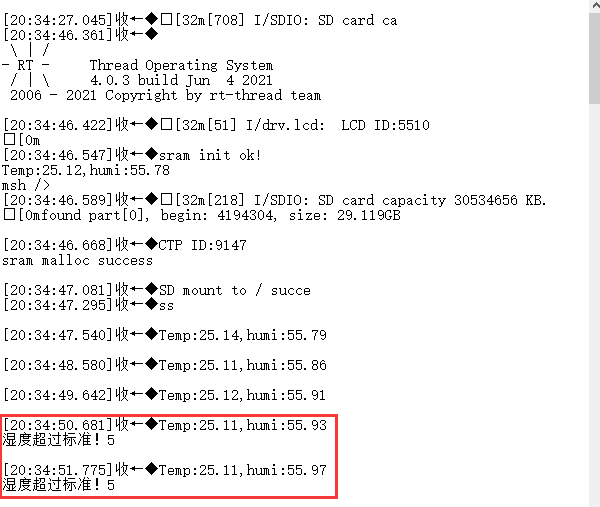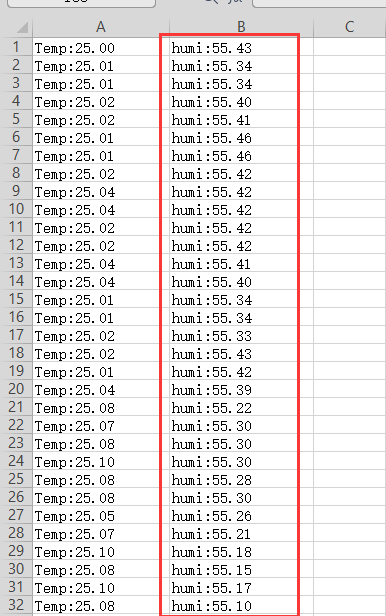目录